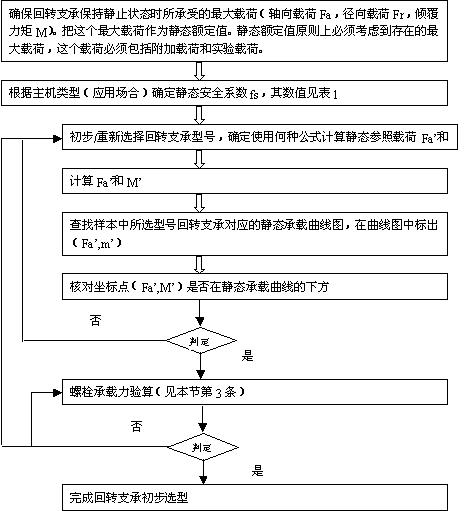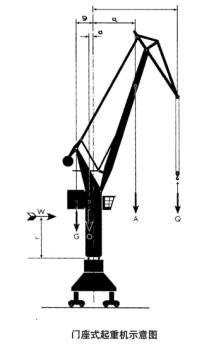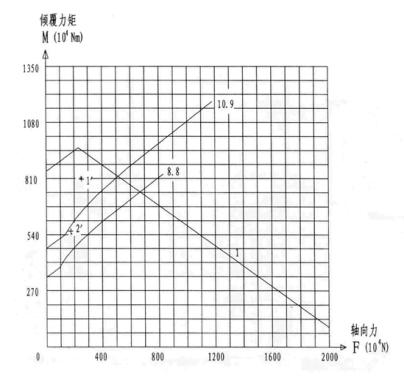• 宜陽龍馬軸承有限公司
• 地　址：河南省洛陽市宜陽軸承産業園區
• 銷售部：0379-65216522　13703888536
• 技術部：0379-65216525　18736312885
• 供應部：0379-65216523　13383895999

# 回轉支承型號選擇計算方法

###### [ 发布日期：2014-12-26 点击：3291 来源:宜陽龍馬軸承有限公司 【打印此文】【關閉窗口】]

靜態選型

1 ）选型计算流程图2 ）静态参照载荷 Fa' 和 M' 的计算方法：

·單排四點接觸球式：

I、a=45° II、a=60°

Fa'=(1.225*Fa+2.676*Fr)*fs Fa'=(Fa+5.046*Fr)*fs

M'=1.225*M*fs M'=M*fs

·單排交叉滾柱式

Fa'=(Fa+2.05Fr)*fs

M'=M*fs

·雙排異徑球式

Fa'=Fa*fs

M'=M*fs

·三排滾柱式

Fa'=Fa*fs

M'=M*fs

·動態選型

·螺栓承載力驗算：

·把回转支承所承受的载荷（没有乘静态安全系数 fs ）作为选择螺栓的载荷。

·查對載荷是否在所需等級螺栓極限負荷曲線以下；

·若螺栓承載能力不夠，可重新選擇回轉支承，或與我公司聯系。

·选型计算距离 - 門坐式起重機（抓鬥）·計八級風力的工作載荷

2) 不计风力，考虑 25% 实验负荷的载荷

Q=260KN lmax=23m

A=75KN amax=11m

O=450KN o=0.75m

G=900KN g=3m

W=27KN r=6.5m

·八級風力時的工作載荷：

Fa=Q+A+O+G=260+75+450+900=1685KN

M=Q*lmax+A*amax+W*r-O*o-G*g=260*23+75*11+27*6.5-450*0.75-900*3=3943KNm

2) 不计风力，考虑 25% 试验负载时的工作载荷

Fa=1.25Q+A+O+G=325+75+450+900=1750KN

M=1.25Q*lmax+A*amax-O*o-G*g=325*23+75*11-45*0.75-900*3=5566.3KNm

·不計風力時工作載荷

Fa=1685KN

M=Q*lmax+A*amax-O*o-G*g=260*23+75*11-450*0.75-900*3=3767.5KNm

Fa'=1750KN*1.45=2537.5KN

M'=5566.3KNm*1.45=8071.1KNm

Fa=1750KN

M=5566.3KNM?

l 线为静态承载能力曲线

8.8 、 10.9 为螺栓承载曲线

1'- 静态载荷点

2'- 螺栓载荷点

1' 点再滚道静态承载曲线 1 下方，因此满足要求

2' 点在 10.9 级螺栓承载曲线下方，因此选择 0.9 级螺栓可以满足要求。